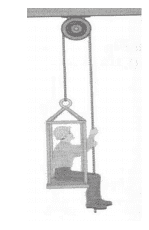# A man pulls himself up;What force does he exert on the elevator?

## Homework StatementA man pulls himself up, the acceleration is 1 m/s², the man's mass is 60 kg and the elevator's mass is 40 kg.
a) Calculate the tension in the rope.
b) Calculate the force that the man exerts on elevator's floor.

Fr = Ma

## The Attempt at a Solution

I calculated letter a) correctly, and found 550N. My problem is on letter b).

Here are my thoughts: The man's free body diagram on the elevator would be: His weigh down, and the elevator's normal (opposing to the force he exerts by newton's 3rd law) up, the difference of those would accelerate him, so, in symbols:

$N_{elevator} - P_{man} = m_{man}a\\\\ a = 1\frac{m}{s^2}; P_{man} = 588 N;\\ N_{elevator} = 588 + 60 = 648N$

However, the answer is supposed to be 110 N. I also noticed that 648N - 550 N is almost equal to 110N, however that doesn't make much sense, since there are two tensions acting on him, and not only one.

PhanthomJay
Homework Helper
Gold Member

## Homework Statement

View attachment 54653
A man pulls himself up, the acceleration is 1 m/s², the man's mass is 60 kg and the elevator's mass is 40 kg.
a) Calculate the tension in the rope.
b) Calculate the force that the man exerts on elevator's floor.

Fr = Ma

## The Attempt at a Solution

I calculated letter a) correctly, and found 550N. My problem is on letter b).

Here are my thoughts: The man's free body diagram on the elevator would be: His weigh down, and the elevator's normal (opposing to the force he exerts by newton's 3rd law) up, the difference of those would accelerate him, so, in symbols:

$N_{elevator} - P_{man} = m_{man}a\\\\ a = 1\frac{m}{s^2}; P_{man} = 588 N;\\ N_{elevator} = 588 + 60 = 648N$

However, the answer is supposed to be 110 N. I also noticed that 648N - 550 N is almost equal to 110N, however that doesn't make much sense, since there are two tensions acting on him, and not only one.

When you draw the FBD of the man, yes, the normal force of the floor on the man acts up, and his weight acts down, but there is tension acting on him also, but if you look closely, is it one or 2 tensions acting on him?

I suppose it's one, but I don't understand why. I see the two tensions acting on him (the one that's acting on his hand, and the one acting on top of the elevator).

Ooohhhh... wait. The one acting on the elevator ISN'T acting on him, it's, as the name implies acting on the elevator. The only tension acting on the man is the one acting on his hand then.

Is this correct?

PhanthomJay
Homework Helper
Gold Member
I suppose it's one, but I don't understand why. I see the two tensions acting on him (the one that's acting on his hand, and the one acting on top of the elevator).

Ooohhhh... wait. The one acting on the elevator ISN'T acting on him, it's, as the name implies acting on the elevator. The only tension acting on the man is the one acting on his hand then.

Is this correct?
Yes, correct. When you look at the FBD of the man-elevator system, there are 2 tensions acting on the system, which I gather you realized by obtaining the corect solution to part a. But only one on the man and the other on the elevator. Drawing the FBD of the man only allows you to calculate the normal force of the elevator on the man, and the normal force of the man on the elevator can be found from Newton's 3rd law. As a check, you may want to draw a FBD of the elevator alone to obtain the Normal force of the man on the elevator directly, although this may be a bit more difficult to visualize, but worth a look.

CAF123
Gold Member
I suppose it's one, but I don't understand why. I see the two tensions acting on him (the one that's acting on his hand, and the one acting on top of the elevator).

Ooohhhh... wait. The one acting on the elevator ISN'T acting on him, it's, as the name implies acting on the elevator. The only tension acting on the man is the one acting on his hand then.

Is this correct?

For part b), you are considering only the forces acting on the man ( he is your system here) so that is why there is only one tension acting on the man. In part a), you considered the system man + elevator so there you had two tensions.

In your opening post, you said that his weight and normal force constitute a third law force pair. That is not right because both these forces act on the same body.

Thanks a lot to you both! It's very clear to me now. Regarding the Weigh and Normal, I got those confused. I should have said that the Weigh of the man applied on the elevator and the Normal applied on the man constitute an action and reaction pair, right?

CAF123
Gold Member
We have the force of earth onto man (gravitational force) and force of man onto earth. This is a third law pair since the two forces act on different bodies. The other third law pair is the force from the elevator on man (normal force) and the force from man onto elevator. (as PhanthomJay mentioned).

The 'Weight of man on elevator' you refer to is (I think it is what you mean) this force from man on elevator in my example above.

PhanthomJay